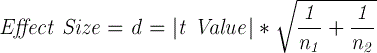## Friday, May 30, 2014

### 2-Sample Pooled t-Test – Effect Size in Excel 2010 and Excel 2013

This is one of the following eight articles on 2-Independent-Sample Pooled t-Tests in Excel

2-Independent-Sample Pooled t-Test in 4 Steps in Excel 2010 and Excel 2013

Excel Variance Tests: Levene’s, Brown-Forsythe, and F Test For 2-Sample Pooled t-Test in Excel 2010 and Excel 2013

Excel Normality Tests Kolmogorov-Smirnov, Anderson-Darling, and Shapiro Wilk Tests For Two-Sample Pooled t-Test

Two-Independent-Sample Pooled t-Test - All Excel Calculations

2-Sample Pooled t-Test – Effect Size in Excel 2010 and Excel 2013

2-Sample Pooled t-Test Power With G*Power Utility

Mann-Whitney U Test in 12 Steps in Excel as 2-Sample Pooled t-Test Nonparametric Alternative in Excel 2010 and Excel 2013

2- Sample Pooled t-Test = Single-Factor ANOVA With 2 Sample Groups

# Effect Size of a Two- Sample Pooled t-Test in Excel

Effect size in a t-Test is a convention of expressing how large the difference between two groups is without taking into account the sample size and whether that difference is significant.

Effect size of Hypotheses Tests of Mean is usually expressed in measures of Cohen’s d. Cohen’s d is a standardized way of quantifying the size of the difference between the two groups. This standardization of the size of the difference (the effect size) enables classification of that difference in relative terms of “large,” “medium,” and “small.”

A large effect would be a difference between two groups that is easily noticeable with the measuring equipment available. A small effect would be a difference between two groups that is not easily noticed.

Effect size for a two-independent-sample, pooled t-Test is a method of expressing the distance between the difference between sample mean, x_bar1-x_bar2, and the Constant in a standardized form that does not depend on the sample size.

Remember that the Test Statistic (the t Value) for a two-independent-sample t-Test calculated by the following formula:(Click On Image To See a Larger Version)

which equals(Click On Image To See a Larger Version)

since(Click On Image To See a Larger Version)(Click On Image To See a Larger Version)

Since degrees of freedom for a two-independent-sample, pooled t-Test equals the following:

df = n1 + n2 – 2(Click On Image To See a Larger Version)

The t Value specifies the number of Standard Errors that the differences between sample means, x_bar1-x_bar2, is from the Constant. The t Value is dependent upon the sample size, n. The t Value determines whether the test has achieved statistical significance and is dependent upon sample size. Achieving statistical significance means that the Null Hypothesis (H0: x_bar1-x_bar2 = Constant = 0) has been rejected.

The t Value for a two-independent-sample, pooled is calculated as follows:(Click On Image To See a Larger Version)

The Effect Size, d, for a two-independent-sample, pooled t-Test is a very similar measure that does not directly depend on sample size and has the following formula:(Click On Image To See a Larger Version)

spooled pools the sample standard deviations based upon the proportion of combined samples that each of the sample sizes n1 and n2 represent and not the absolute values of n1 and n2. spooled is therefore not directly dependent on sample sizes n1 and n2.

A test’s Effect Size can be quite large even though the test does not achieve statistical significance due to small sample size.

If the t Value has already been calculated, the Effect Size can be quickly calculated by the following formula:(Click On Image To See a Larger Version)

The d measured here is Cohen’s d for a two-independent-sample, pooled t-Test. The Effect Size is a standardized measure of size of the difference that the t-Test is attempting to detect. The Effect Size for a two-independent-sample, pooled t-Test is a measure of that difference in terms of the number of sample standard deviations. Note that sample size has no effect on Effect Size. Effect size values for the two-independent-sample, pooled t-Test are generalized into the following size categories:

d = 0.2 up to 0.5 = small Effect Size

d = 0.05 up to 0.8 = medium Effect Size

d = 0.8 and above = large Effect Size

In this example, the Effect Size is calculated as follows:

d = |x_bar1 - x_bar2 – Constant| / spooled = |43.56 – 33.53 – 0| / 16.09 = 0.623

An Effect Size of d = 0.623 is considered to be a medium effect.

Excel Master Series Blog Directory

Statistical Topics and Articles In Each Topic

• Histograms in Excel
• Bar Chart in Excel
• Combinations & Permutations in Excel
• Normal Distribution in Excel
• t-Distribution in Excel
• Binomial Distribution in Excel
• z-Tests in Excel
• t-Tests in Excel
• Hypothesis Tests of Proportion in Excel
• Chi-Square Independence Tests in Excel
• Chi-Square Goodness-Of-Fit Tests in Excel
• F Tests in Excel
• Correlation in Excel
• Pearson Correlation in Excel
• Spearman Correlation in Excel
• Confidence Intervals in Excel
• Simple Linear Regression in Excel
• Multiple Linear Regression in Excel
• Logistic Regression in Excel
• Single-Factor ANOVA in Excel
• Two-Factor ANOVA With Replication in Excel
• Two-Factor ANOVA Without Replication in Excel
• Randomized Block Design ANOVA in Excel
• Repeated-Measures ANOVA in Excel
• ANCOVA in Excel
• Normality Testing in Excel
• Nonparametric Testing in Excel
• Post Hoc Testing in Excel
• Creating Interactive Graphs of Statistical Distributions in Excel
• Solving Problems With Other Distributions in Excel
• Optimization With Excel Solver
• Chi-Square Population Variance Test in Excel
• Analyzing Data With Pivot Tables and Pivot Charts
• SEO Functions in Excel
• Time Series Analysis in Excel
• VLOOKUP
• Simplifying Useful Excel Functions